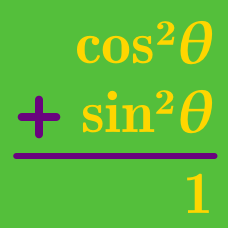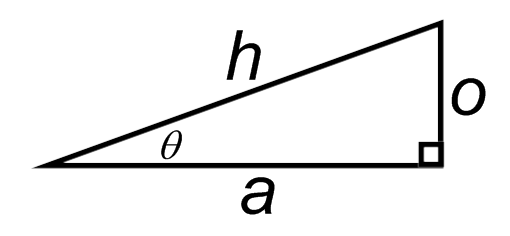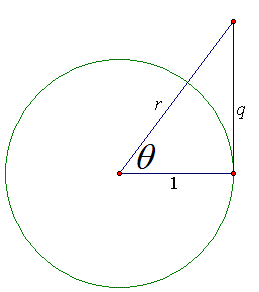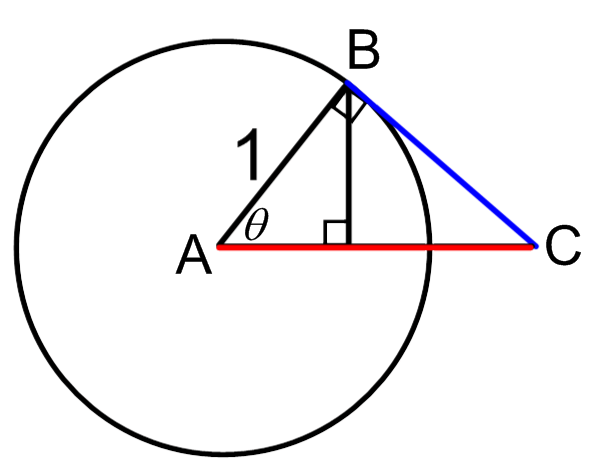Geometry

# Visualizing Pythagorean IdentitiesThe diagram above implies $$a^2 + o^2 = h^2$$. What Pythagorean identity is derived when both sides of the equal sign are divided by $$o^2$$?

Which of these equations cannot be derived from the others?Which of the following is equivalent to $$r ?$$Which identity is directly implied by applying the Pythagorean Theorem to triangle $$ABC?$$

Remember: the Pythagorean Theorem says that $$AB^2 + BC^2 = AC^2.$$

Which identity can be obtained by dividing both sides of $$\sin^2(\theta) + \cos^2(\theta) = 1$$ by $$\cos^2(\theta) ?$$

×